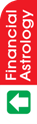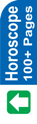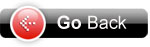Astrology Software
Indian AstrologyCalculation of Longitudes of Planets Now to place the planets in the horoscopes, longitudes of planets havae to be calculated. For this, we proceed as under: 1. Refer to Annual Ephemeries, 1993. Longitudes of different planets for the entire year, month-wise, day-wise, have been given as at 5.30 AM IST. On page 30, search the required date, i.e. 10th December. Against this the longitudes for planets on 10.12.1993 at 5.30 AM are given. But our required birth time is 3.50 AM, i.e. 22h 20 m after 9.12.1993 at 5.30 AM. 2. Find out the longitudes of planets as on 9.12.1993 at 5.30 AM from page 30, and add the distance covered by planets in 22 h 20 m. This can be done in the following way : Find out the longitudes of planets as given below : ---------------------------------------------------------------------------------------------------------- 9.12.1993 10.12.1993 Difference ---------------------------------------------------------------------------------------------------------- s ° ' s ° ' ---------------------------------------------------------------------------------------------------------- Sun 7 - 23 - 08 7 - 24 - 09 1° 1' Moon 5 - 24 - 07 6 - 8 - 36 14° 29' Mars 7 - 27 - 58 7 - 28 - 43 45' Mercury 7 - 9 - 10 7 - 10 - 41 1° 31' Jupiter 6 - 12 - 01 6 - 12 - 12 11' Venus 7 - 13 - 46 7 - 15 - 02 1° 16' Saturn 10- 1 - 20 10- 1 - 24 4' Rahu 7 - 9 - 12 7 - 9 - 14 2' Ketu 1 - 9 - 12 1 - 9 - 14 2' ---------------------------------------------------------------------------------------------------------- Calculation of Longitude of Sun Now we would use the proportional logarithms tables provided in the Annual Ephemeries, 1993 in the end. Horizontally, degrees or hours are given, and vertically, minutes are given. As calculated above, speed of Sun comes to 1° 1'. Log for 1° 1' (From the above Table) 1.3730 Add log for the difference of 22 h 20 m 0.0313 (Take horizontally 22 h and vertically 20', and see them meet at 0313) 1.4043 Now, search this figure (1.4043) in the above Table. The nearest figure is 1.4025. This is written at the cross-section of 0° 57'. This means Sun has travelled 57' in 22 h 20'. Longitude of Sun on 9.12.1993 7 s 23° 08' at 5.30 AM Add the distance covered in 0 s 00° 57' 22 hr 20 m Longitude of Sun at the given birth time 7s 24° 05' Calculation of Longitude of Moon As calculated above, speed of Moon comes to 14° 29'. Log for 14° 27'' (From the above Table) 0.2193 Add log for the difference of 22 h 20 m 0.0313 (Take horizontally 22 h and vertically 20', and see them meet at 0313) 0.2506 Now, search this figure (0.2506) in the above Table. The nearest figure is 0.2504. This is written at the cross-section of 13° 29' . This means Moon has travelled 13° 29' in 22 h 20'. Longitude of Moon on 9.12.1993 5 s 24° 07' at 5.30 AM Add the distance covered in 0 s 13° 29' 22 hr 20 m Longitude of Moon at the given birth time 6s 07° 36' In the case of Moon, exact longitude of Moon is required, as with its help Vimshottari Dasha will be calculated. A little approximate longitude will increase or decrease the number of days in Vimshottari Dasha. In this case, we have taken the nearest figure of 0.2504. The next to it is 0.2509. Distance for 0.2504 = 13° 29' Distance for 0.2509 = 13° 28' Therefore, difference for 0.0005 = 1' = 60 s. Difference for 0.0002 = 60/.0005 x 0.0002 = 24 s. We had calculated the distance as 13° 29', whereas it should actually be 24 s less. Hence, the actual distance should be 13° 28' 36 s. Add this to 5s 24° 07'. It comes to 6s 7° 35' 36". You would see that approximately, it is equal to 6s 7° 36'. But this accurate figure is important while calculating Vimshottari Dasha. Calculation of Longitude of Mars As calculated above, speed of Mars comes to 45'. Log for 45' (From the above Table) 1.5051 Add log for the difference of 22 h 20 m 0.0313 (Take horizontally 22 h and vertically 20', and see them meet at 0313) 1.5364 Now, search this figure (1.5364) in the above Table. The nearest figure is 1.5351. This is written at the cross-section of 42' . This means Mars has travelled 42' in 22 h 20'. Longitude of Mars on 9.12.1993 7 s 27° 58' at 5.30 AM Add the distance covered in 0 s 00° 42' 22 hr 20 m Longitude of Mars at the given birth time 7s 28° 40'   Calculation of Longitude of Mercury As calculated above, speed of Mercury comes to 1° 30'. Log for 1° 30'' (From the above Table) 1.1993 Add log for the difference of 22 h 20 m 0.0313 (Take horizontally 22 h and vertically 20', and see them meet at 0313) 1.2306 Now, search this figure (1.2306) in the above Table. The nearest figure is 1.2341. This is written at the cross-section of 1° 24' . This means Mercury has travelled 1° 24' in 22 h 20'. Longitude of Mercury on 9.12.1993 7 s 09° 10' at 5.30 AM Add the distance covered in 0 s 01° 24' 22 hr 20 m Longitude of Mercury at the given birth time 7s 10° 34' Calculation of Longitude of Jupiter As calculated above, speed of Jupiter comes to 11'. Log for 11' (From the above Table) 2.1170 Add log for the difference of 22 h 20 m 0.0313 (Take horizontally 22 h and vertically 20', and see them meet at 0313) 2.1483 Now, search this figure (2.1483) in the above Table. The nearest figure is 2.1584. This is written at the cross-section of 10' . This means Jupiter has travelled 10' in 22 h 20'. Longitude of Jupiter on 9.12.1993 6 s 12° 01' at 5.30 AM Add the distance covered in 0 s 00° 10' 22 hr 20 m Longitude of Jupiter at the given birth time 6s 12° 11' Calculation of Longitude of Venus As calculated above, speed of Venus comes to 1° 16'. Log for 1° 16' (From the above Table) 1.2775 Add log for the difference of 22 h 20 m 0.0313 (Take horizontally 22 h and vertically 20', and see them meet at 0313) 1.3088 Now, search this figure (1.3088) in the above Table. The nearest figure is 1.3071. This is written at the cross-section of 1° 11' . This means Venus has travelled 1° 11' in 22 h 20'. Longitude of Venus on 9.12.1993 7 s 13° 46' at 5.30 AM Add the distance covered in 0 s 01° 11' 22 hr 20 m Longitude of Venus at the given birth time 7s 14° 57' Calculation of Longitude of Saturn As calculated above, speed of Saturn comes to 04'. Log for 04' (From the above Table) 2.5563 Add log for the difference of 22 h 20 m 0.0313 (Take horizontally 22 h and vertically 20', and see them meet at 0313) 2.5876 Now, search this figure (2.5876) in the above Table. The nearest figure is 2.5563. This is written at the cross-section of 04' . This means Saturn has travelled 04' in 22 h 20'. Longitude of Saturn on 9.12.1993 10 s 01° 20' at 5.30 AM Add the distance covered in 0 s 00° 04' 22 hr 20 m Longitude of Saturn at the given birth time 10s 01° 24' Calculation of Longitude of Rahu As calculated above, speed of Rahu comes to 02'. Log for 02' (From the above Table) 2.8573 Add log for the difference of 22 h 20 m 0.0313 (Take horizontally 22 h and vertically 20', and see them meet at 0313) 2.8886 Now, search this figure (2.8886) in the above Table. The nearest figure is 2.8573. This is written at the cross-section of 02' . This means Rahu has travelled 02' in 22 h 20'. Longitude of Rahu on 9.12.1993 7 s 09° 12' at 5.30 AM Add the distance covered in 0 s 00° 02' 22 hr 20 m Longitude of Rahu at the given birth time 7s 09° 14' Calculation of Longitude of Ketu As calculated above, speed of Ketu comes to 02'. Log for 02' (From the above Table) 2.8573 Add log for the difference of 22 h 20 m 0.0313 (Take horizontally 22 h and vertically 20', and see them meet at 0313) 2.8886 Now, search this figure (2.8886) in the above Table. The nearest figure is 2.8573. This is written at the cross-section of 02' . This means Ketu has travelled 02' in 22 h 20'. Longitude of Ketu on 9.12.1993 1 s 09° 12' at 5.30 AM Add the distance covered in 0 s 00° 02' 22 hr 20 m Longitude of Ketu at the given birth time 1s 09° 14' To sum up, longitudes of different planets and Ascendant are: Ascendant : 6 s 10° 28' Sun : 7 s 24° 05' Moon : 6 s 07° 36' Mars : 7 s 28° 40' Mercury : 7 s 10° 34' Jupiter : 6 s 12° 11' Venus : 7 s 14° 57' Saturn : 10s 01° 24' Rahu : 7 s 09° 14' Ketu : 1 s 09° 14' Nowadays computer is being used for calculating horoscopes. It is more accurate and easy to use. If you calculate the longitudes of planets in the above case with the help of a computer, you would find marginal differences which is the result of accurate calculations.Astrowealth Astrology Software Software for Website Astrology Reports Free Services Regional Language Important Link